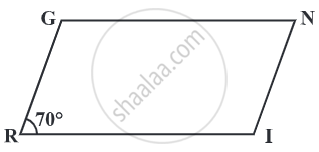# In a parallelogram RING, if m∠R = 70°, find all the other angles. - Mathematics

Sum
In a parallelogram RING, if m∠R = 70°, find all the other angles.#### Solution

Given: m∠R = 70°
Then, m∠N = 70°
because ∠R and ∠N are opposite angles of a parallelogram.
Since ∠R and ∠I are supplementary,
m∠I = 180° – 70° = 110°
Also, m∠G = 110° since ∠G is opposite to ∠I
Thus, m∠R = m∠N = 70° and m∠I = m∠G = 110°.
Is there an error in this question or solution?

Share